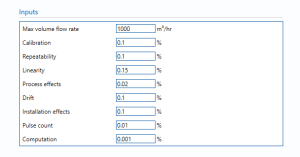# Liquid Ultrasonic Meter

## InputsMax volume flow rate – the maximum flow rate that the liquid passing through the USM.

Calibration – Percentage uncertainty in calibration of the USM.

Repeatability – Percentage uncertainty in the reputability of the reading from the USM.

Linearity – The percentage uncertainty due to linearity. Linearity is calculated by getting the average and the range of the measurements made. The sample averages and ranges will be used with the reference values to determine linearity either graphically or by calculations

Process effects – Additional uncertainty due to any unaccounted effects in pressure, temperature, viscosity of liquid passing through the USM.

Drift – Additional uncertainty allowance due to meter drift. Normally left at zero, unless evidence is present to suggest a higher value.

Installation effects – The percentage uncertainty due to installation effects. Installation effects can be from several sources such as a distorted swirl in the profile of the flow due to the layout of the piping system.

Pulse count – Uncertainty due to number of pulses counted. Negligible term included for completeness, assume 0.01%.

Computation – Uncertainty due to computational errors in flow computer.

## Uncertainty Budget

The uncertainty budget table shows a break down of the different components that contribute to the overall calculated uncertainty.The values input into the uncertainty budget are derived from the measured pressure and the transmitter specific values relating to its calibration and specification. These values are taken in as the expanded uncertainties and are divided by a coverage factor to gain the standard uncertainty.

The coverage factor is determined by the probability distribution that best suits that uncertainty component. The standard uncertainty is then multiplied by the sensitivity value then squared. This is done for each component that contributes to the overall uncertainty in volume flow rate. The Standard Uncertainty in the volume flow rate is the square root of the sum of each component variance as shown in the following equation:### Calculated UncertaintyThe Expanded Uncertainty is the Standard Uncertainty multiplied by the coverage factor (k). The coverage factor is defaulted to k = 2 (equivalent to a confidence level of approximately 95%).

The Relative Uncertainty is the Expanded Uncertainty divided by the volume flow rate.

Back to Uncertainty Modules